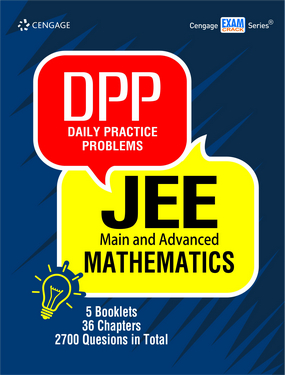###### Home
>

Test Prep### Daily Practice Problems JEE Main and Advanced: Mathematics

Author(s): Cengage India

ISBN: 9789353501518

Edition: 1st

#### Rs. 480

Binding: Paperback

Pages: 524

Trim Size : 279 x 216 mm

Daily Practice Problems (DPPs) are a set of 5 booklets of Mathematics that contain questions segregated chapterwise and topicwise. The questions have been carefully picked and set by authors understanding the need of a JEE aspirant as per their daily preparation schedule. Each chapter in a booklet is presented as a DPP containing questions of both JEE Main and Advanced level. The question types used in these DPPs are in exact lines with those that appear in JEE Main and Advanced. Detailed solutions are given to all the questions at the end of each booklet.

• A total of 2700 questions are included in 5 booklets.
• The question bank caters to both JEE Main and Advanced preparation.
• Four types of questions have been included in the DPPs – Subjective Type, Single Correct Answer Type, Multiple Correct Answers Type and Linked Comprehension Type.
• Solutions to all questions are given at the end of each booklet.

Algebra

Chapter 1: Inequalities and Modulus

Chapter 2: Theory of Equations

Chapter 3: Complex Numbers

Chapter 4: Progression and Series

Chapter 5: Inequalities Involving Means

Chapter 6: Permutation and Combination

Chapter 7: Binomial Theorem

Chapter 8: Determinants

Chapter 9: Matrices

Chapter 10: Probability

Calculus

Chapter 1: Functions

Chapter 2: Limits

Chapter 3: Continuity and Differentiability

Chapter 4: Methods of Differentiation

Chapter 5: Applications of Derivatives

Chapter 6: Monotonicity and Maxima–Minima of Functions

Chapter 7: Indefinite Integration

Chapter 8: Definite Integration

Chapter 9: Area

Chapter 10: Differential Equations

Coordinate Geometry

Chapter 1: Coordinate System

Chapter 2: Straight Lines

Chapter 3: Pair of Straight Lines

Chapter 4: Circle

Chapter 5: Parabola

Chapter 6: Ellipse

Chapter 7: Hyperbola

Trigonometry

Chapter 1: Logarithm and Its Applications

Chapter 2: Trigonometric Functions

Chapter 3: Trigonometric Ratios for Compound, Multiple, Sub-multiple Angles and Transformation Formulas

Chapter 4: Trigonometric Equations

Chapter 5: Inverse Trigonometric Functions

Chapter 6: Solutions and Properties of Triangle

Vectors and 3D Geometry

Chapter 1: Introduction to Vectors

Chapter 2: Different Products of Vectors and Their Geometrical Applications

Chapter 3: Three-Dimensional Geometry